JAJSCI4 September   2016

PRODUCTION DATA.

1. 特長
2. アプリケーション
3. 概要
4. 改訂履歴
5. 概要（続き）
6. Pin Configuration and Function
7. Specifications
8. Parameter Measurement Information
9. Detailed Description
10. 10Application and Implementation
1. 10.1 Application Information
2. 10.2 Typical Applications
11. 11Power Supply Recommendations
12. 12Layout
13. 13デバイスおよびドキュメントのサポート
14. 14メカニカル、パッケージ、および注文情報

#### パッケージ・オプション

デバイスごとのパッケージ図は、PDF版データシートをご参照ください。

• DW|16

## 7 Specifications

### 7.1 Absolute Maximum Ratings

Over operating free-air temperature range (unless otherwise noted)(1)
MIN MAX UNIT
VCC1 Supply-voltage input side GND1 – 0.3 6 V
VCC2 Positive supply-voltage output side (VCC2 – GND2) –0.3 35 V
VEE2 Negative supply-voltage output side (VEE2 – GND2) –17.5 0.3 V
V(SUP2) Total-supply output voltage (VCC2 - VEE2) –0.3 35 V
V(OUTH) Positive gate-driver output voltage VEE2 – 0.3 VCC2 + 0.3 V
V(OUTL) Negative gate-driver output voltage VEE2 – 0.3 VCC2 + 0.3 V
I(OUTH) Gate-driver high output current Maximum pulse width = 10 μs, Maximum duty cycle = 0.2%) 2.7 A
I(OUTL) Gate-driver low output current Maximum pulse width = 10 μs, Maximum duty cycle = 0.2%) 5.5 A
V(LIP) Voltage at IN+, IN–,FLT, RDY, RST GND1 – 0.3 VCC1 + 0.3 V
I(LOP) Output current of FLT, RDY 10 mA
V(DESAT) Voltage at DESAT GND2 – 0.3 VCC2 + 0.3 V
V(CLAMP) Clamp voltage VEE2 – 0.3 VCC2 + 0.3 V
TJ Junction temperature –40 150 °C
TSTG Storage temperature –65 150 °C
(1) Stresses beyond those listed under Absolute Maximum Ratings may cause permanent damage to the device. These are stress ratings only, which do not imply functional operation of the device at these or any other conditions beyond those indicated under Recommended Operating Conditions. Exposure to absolute-maximum-rated conditions for extended periods may affect device reliability.

### 7.2 ESD Ratings

VALUE UNIT
V(ESD) Electrostatic discharge Human-body model (HBM), per AEC Q100-002(1) ±4000 V
Charged-device model (CDM), per AEC Q100-011 ±1500
(1) AEC Q100-002 indicates that HBM stressing shall be in accordance with the ANSI/ESDA/JEDEC JS-001 specification.

### 7.3 Recommended Operating Conditions

over operating free-air temperature range (unless otherwise noted)
MIN NOM MAX UNIT
VCC1 Supply-voltage input side 2.25 5.5 V
VCC2 Positive supply-voltage output side (VCC2 – GND2) 15 30 V
V(EE2) Negative supply-voltage output side (VEE2 – GND2) –15 0 V
V(SUP2) Total supply-voltage output side (VCC2 – VEE2) 15 30 V
V(IH) High-level input voltage (IN+, IN–, RST) 0.7 × VCC1 VCC1 V
V(IL) Low-level input voltage (IN+, IN–, RST) 0 0.3 × VCC1 V
tUI Pulse width at IN+, IN– for full output (CLOAD = 1 nF) 40 ns
tRST Pulse width at RST for resetting fault latch 800 ns
TA Ambient temperature –40 125 °C

### 7.4 Thermal Information

THERMAL METRIC(1) ISO5852S-Q1 UNIT
DW (SOIC)
16 PINS
RθJA Junction-to-ambient thermal resistance 99.6 °C/W
RθJC(top) Junction-to-case (top) thermal resistance 48.5 °C/W
RθJB Junction-to-board thermal resistance 56.5 °C/W
ψJT Junction-to-top characterization parameter 29.2 °C/W
ψJB Junction-to-board characterization parameter 56.5 °C/W
(1) For more information about traditional and new thermal metrics, see the Semiconductor and IC Package Thermal Metrics application report.

### 7.5 Power Ratings

Full-chip power dissipation is derated 10.04 mW/°C beyond 25°C ambient temperature. At 125°C ambient temperature, a maximum of 251 mW total power dissipation is allowed. Power dissipation can be optimized depending on ambient temperature and board design, while ensuring that the junction temperature does not exceed 150°C.
PARAMETER TEST CONDITIONS MIN TYP MAX UNIT
PD Maximum power dissipation (both sides) VCC1 = 5.5-V, VCC2 = 30-V, TA = 25°C 1255 mW
PID Maximum input power dissipation VCC1 = 5.5-V, VCC2 = 30-V, TA = 25°C 175 mW
POD Maximum output power dissipation VCC1 = 5.5-V, VCC2 = 30-V, TA = 25°C 1080 mW

### 7.6 Insulation Specifications

PARAMETER TEST CONDITIONS VALUE UNIT
GENERAL
CLR External clearance(1) Shortest terminal-to-terminal distance through air 8 mm
CPG External creepage(1) Shortest terminal-to-terminal distance across the package surface 8 mm
DTI Distance through the insulation Minimum internal gap (internal clearance) 21 µm
CTI Comparative tracking index DIN EN 60112 (VDE 0303-11); IEC 60112; Material Group I according to IEC 60664-1; UL 746A >600 V
Material group I
Overvoltage Category Rated mains voltage ≤ 600 VRMS I-IV
Rated mains voltage ≤ 1000 VRMS I-III
DIN V VDE V 0884-10 (VDE V 0884-10):2006-12(2)
VIORM Maximum repetitive peak isolation voltage AC voltage (bipolar) 2121 VPK
VIOWM Maximum isolation working voltage AC voltage (sine wave) Time dependent dielectric breakdown (TDDB) test, see Figure 1 1500 VRMS
DC voltage 2121 VDC
VIOTM Maximum transient isolation voltage VTEST = VIOTM; t = 60 s (qualification); t = 1 s (100% production) 8000 VPK
VIOSM Maximum surge isolation voltage(3) Test method per IEC 60065, 1.2/50 µs waveform, VTEST = 1.6 × VIOSM = 12800 VPK (qualification) 8000
qpd Apparent charge(4) Method a: After I/O safety test subgroup 2/3,
Vini = VIOTM, tini = 60 s;
Vpd(m) = 1.2 × VIORM = 2545 VPK ,
tm = 10 s
≤5 pC
Method a: After environmental tests subgroup 1,
Vini = VIOTM, tini = 60 s;
Vpd(m) = 1.6 × VIORM = 3394 VPK ,
tm = 10 s
≤5
Method b1: At routine test (100% production) and preconditioning (type test)
Vini = VIOTM, tini = 60 s;
Vpd(m) = 1.875× VIORM = 3977 VPK ,
tm = 10 s
≤5
CIO Barrier capacitance, input to output(5) VIO = 0.4 sin (2πft), f = 1 MHz 1 pF
RIO Isolation resistance, input to output(5) VIO = 500 V, TA = 25°C > 1012 Ω
VIO = 500 V, 100°C ≤ TA ≤ 125°C > 1011
VIO = 500 V at TS = 150°C > 109
Pollution degree 2
Climatic category
UL 1577
VISO Withstand isolation voltage VTEST = VISO = 5700 VRMS, t = 60 s (qualification); VTEST = 1.2 × VISO = 6840 VRMS, t = 1 s (100% production) 5700 VRMS
(1) Creepage and clearance requirements should be applied according to the specific equipment isolation standards of an application. Care should be taken to maintain the creepage and clearance distance of a board design to ensure that the mounting pads of the isolator on the printed-circuit board do not reduce this distance. Creepage and clearance on a printed-circuit board become equal in certain cases. Techniques such as inserting grooves and/or ribs on a printed circuit board are used to help increase these specifications.
(2) This coupler is suitable for safe electrical insulation only within the maximum operating ratings. Compliance with the safety ratings shall be ensured by means of suitable protective circuits.
(3) Testing is carried out in air or oil to determine the intrinsic surge immunity of the isolation barrier.
(4) Apparent charge is electrical discharge caused by a partial discharge (pd).
(5) All pins on each side of the barrier tied together creating a two-terminal device

### 7.7 Safety Limiting Values

Safety limiting intends to minimize potential damage to the isolation barrier upon failure of input or output circuitry. A failure of the I/O can allow low resistance to ground or the supply and, without current limiting, dissipate sufficient power to overheat the die and damage the isolation barrier, potentially leading to secondary system failures.
PARAMETER TEST CONDITIONS MIN TYP MAX UNIT
IS Safety input, output, or supply current RθJA = 99.6°C/W, VI = 2.75 V, TJ = 150°C, TA = 25°C, see Figure 2 456 mA
RθJA = 99.6°C/W, VI = 3.6 V, TJ = 150°C, TA = 25°C, see Figure 2 346
RθJA = 99.6°C/W, VI = 5.5 V, TJ = 150°C, TA = 25°C, see Figure 2 228
RθJA = 99.6°C/W, VI = 15 V, TJ = 150°C, TA = 25°C, see Figure 2 84
RθJA = 99.6°C/W, VI = 30 V, TJ = 150°C, TA = 25°C, see Figure 2 42
PS Safety input, output, or total power RθJA = 99.6°C/W, TJ = 150°C, TA = 25°C, see Figure 3 255(1) mW
TS Maximum ambient safety temperature 150 °C
(1) Input, output, or the sum of input and output power should not exceed this value

The safety-limiting constraint is the maximum junction temperature specified in the data sheet. The power dissipation and junction-to-air thermal impedance of the device installed in the application hardware determines the junction temperature. The assumed junction-to-air thermal resistance in the Thermal Information table is that of a device installed on a high-K test board for leaded surface-mount packages. The power is the recommended maximum input voltage times the current. The junction temperature is then the ambient temperature plus the power times the junction-to-air thermal resistance.

### 7.8 Safety-Related Certifications

VDE CSA UL CQC TUV
Certified according to DIN V VDE V 0884-10
(VDE V 0884-10):2006-12 and DIN EN 61010-1 (VDE 0411-1):2011-07
Plan to certify under CSA Component Acceptance Notice 5A, IEC 60950-1, and IEC 60601-1 Recognized under UL 1577 Component Recognition Program Certified according to GB4943.1-2011 Certified according to
EN 61010-1:2010 (3rd Ed) and
EN 60950-1:2006/A11:2009/A1:2010/
A12:2011/A2:2013
Reinforced Insulation Maximum Transient isolation voltage, 8000 VPK;
Maximum surge isolation voltage, 8000 VPK,
Maximum repetitive peak isolation voltage, 2121 VPK
Isolation Rating of 5700 VRMS;
Reinforced insulation per CSA 60950-1- 07+A1+A2 and IEC 60950-1 (2nd Ed.), 800 VRMS max working voltage (pollution degree 2, material group I) ;

2 MOPP (Means of Patient Protection) per CSA 60601-1:14 and IEC 60601-1 Ed. 3.1, 250 VRMS (354 VPK) max working voltage
Single Protection, 5700 VRMS (1) Reinforced Insulation, Altitude ≤ 5000m, Tropical climate, 400 VRMS maximum working voltage 5700 VRMS Reinforced insulation per
EN 61010-1:2010 (3rd Ed) up to working voltage of 600 VRMS

5700 VRMS Reinforced insulation per
EN 60950-1:2006/A11:2009/A1:2010/
A12:2011/A2:2013 up to working voltage of 800 VRMS
Certification completed
Certificate number: 40040142
Certificate planned Certification completed
File number: E181974
Certification completed
Certificate number: CQC16001141761
Certification completed
Client ID number: 77311
(1) Production tested ≥ 6840 VRMS for 1 second in accordance with UL 1577.

### 7.9 Electrical Characteristics

Over recommended operating conditions unless otherwise noted. All typical values are at TA = 25°C, VCC1 = 5 V,
VCC2 – GND2 = 15 V, GND2 – VEE2 = 8 V
PARAMETER TEST CONDITIONS MIN TYP MAX UNIT
VOLTAGE SUPPLY
VIT+(UVLO1) Positive-going UVLO1 threshold-voltage input side (VCC1 – GND1) 2.25 V
VIT-(UVLO1) Negative-going UVLO1 threshold-voltage input side (VCC1 – GND1) 1.7 V
VHYS(UVLO1) UVLO1 Hysteresis voltage (VIT+ – VIT–) input side 0.2 V
VIT+(UVLO2) Positive-going UVLO2 threshold-voltage output side (VCC2 – GND2) 12 13 V
VIT–(UVLO2) Negative-going UVLO2 threshold-voltage output side (VCC2 – GND2) 9.5 11 V
VHYS(UVLO2) UVLO2 hysteresis voltage (VIT+ – VIT–) output side 1 V
IQ1 Input-supply quiescent current 2.8 4.5 mA
IQ2 Output-supply quiescent current 3.6 6 mA
LOGIC I/O
VIT+(IN,RST) Positive-going input-threshold voltage (IN+, IN–, RST) 0.7 × VCC1 V
VIT–(IN,RST) Negative-going input-threshold voltage (IN+, IN–, RST) 0.3 × VCC1 V
VHYS(IN,RST) Input hysteresis voltage (IN+, IN–, RST) 0.15 × VCC1 V
IIH High-level input leakage at (IN+)(1) IN+ = VCC1 100 µA
IIL Low-level input leakage at (IN–, RST)(2) IN– = GND1, RST = GND1 -100 µA
IPU Pullup current of FLT, RDY V(RDY) = GND1, V(FLT) = GND1 100 µA
V(OL) Low-level output voltage at FLT, RDY I(FLT) = 5 mA 0.2 V
GATE DRIVER STAGE
V(OUTPD) Active output pulldown voltage I(OUTH/L) = 200 mA, VCC2 = open 2 V
VOUTH High-level output voltage I(OUTH) = –20 mA VCC2 – 0.5 VCC2 – 0.24 V
VOUTL Low-level output voltage I(OUTL) = 20 mA VEE2 + 13 VEE2 + 50 mV
I(OUTH) High-level output peak current IN+ = high, IN– = low,
V(OUTH) = VCC2 - 15 V
1.5 2.5 A
I(OUTL) Low-level output peak current IN+ = low, IN– = high,
V(OUTL) = VEE2 + 15 V
3.4 5 A
I(OLF) Low-level output current during fault condition 130 mA
ACTIVE MILLER CLAMP
V(CLP) Low-level clamp voltage I(CLP) = 20 mA VEE2 + 0.015 VEE2 + 0.08 V
I(CLP) Low-level clamp current V(CLAMP) = VEE2 + 2.5 V 1.6 2.5 3.3 A
V(CLTH) Clamp threshold voltage 1.6 2.1 2.5 V
SHORT CIRCUIT CLAMPING
V(CLP-OUTH) Clamping voltage
(VOUTH – VCC2)
IN+ = high, IN– = low, tCLP = 10 µs, I(OUTH) = 500 mA 1.1 1.3 V
V(CLP-OUTL) Clamping voltage
(VOUTL – VCC2)
IN+ = high, IN– = low, tCLP = 10 µs, I(OUTL) = 500 mA 1.3 1.5 V
V(CLP-CLP) Clamping voltage
(VCLP – VCC2)
IN+ = high, IN– = low, tCLP = 10 µs, I(CLP) = 500 mA 1.3 V
V(CLP-CLAMP) Clamping voltage at CLAMP IN+ = High, IN– = Low, I(CLP) = 20 mA 0.7 1.1 V
V(CLP-OUTL) Clamping voltage at OUTL
(VCLP – VCC2)
IN+ = High, IN– = Low, I(OUTL) = 20 mA 0.7 1.1 V
DESAT PROTECTION
I(CHG) Blanking-capacitor charge current V(DESAT) – GND2 = 2 V 0.42 0.5 0.58 mA
I(DCHG) Blanking-capacitor discharge current V(DESAT) – GND2 = 6 V 9 14 mA
V(DSTH) DESAT threshold voltage with respect to GND2 8.3 9 9.5 V
V(DSL) DESAT voltage with respect to GND2, when OUTH or OUTL is driven low 0.4 1 V
(1) IIH for IN–, RST pin is zero as they are pulled high internally
(2) IIL for IN+ is zero, as it is pulled low internally

### 7.10 Switching Characteristics

Over recommended operating conditions unless otherwise noted. All typical values are at TA = 25°C, VCC1 = 5 V,
VCC2 – GND2 = 15 V, GND2 – VEE2 = 8 V
PARAMETER TEST CONDITIONS MIN TYP MAX UNIT
tr Output-signal rise time at OUTH CLOAD = 1 nF See Figure 44, Figure 45, and Figure 46 12 18 35 ns
tf Output-signal fall time at OUTL CLOAD = 1 nF 12 20 37 ns
tPLH, tPHL Propagation Delay CLOAD = 1 nF 76 110 ns
tsk-p Pulse skew |tPHL – tPLH| CLOAD = 1 nF 20 ns
tsk-pp Part-to-part skew CLOAD = 1 nF 30(1) ns
tGF (IN,/RST) Glitch filter on IN+, IN–, RST CLOAD = 1 nF 20 30 40 ns
tDS (90%) DESAT sense to 90% VOUTH/L delay CLOAD = 10 nF 553 760 ns
tDS (10%) DESAT sense to 10% VOUTH/L delay CLOAD = 10 nF 2 3.5 μs
tDS (GF) DESAT-glitch filter delay CLOAD = 1 nF 330 ns
tDS (FLT) DESAT sense to FLT-low delay See Figure 46 1.4 μs
tLEB Leading-edge blanking time See Figure 44 and Figure 45 310 400 480 ns
tGF(RSTFLT) Glitch filter on RST for resetting FLT 300 800 ns
CI Input capacitance(2) VI = VCC1 / 2 + 0.4 × sin (2πft), f = 1 MHz, VCC1 = 5 V 2 pF
CMTI Common-mode transient immunity VCM = 1500 V, see Figure 47 100 120 kV/μs
(1) Measured at same supply voltage and temperature condition
(2) Measured from input pin to ground.

### 7.11 Safety and Insulation Characteristics Curves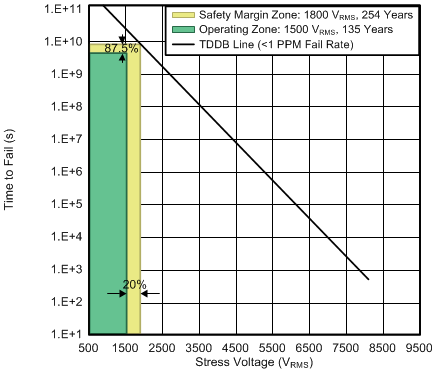TA upto 150°C Stress-voltage frequency = 60 Hz
Figure 1. Reinforced High-Voltage Capacitor Lifetime ProjectionFigure 2. Thermal Derating Curve for Safety Limiting Current per VDE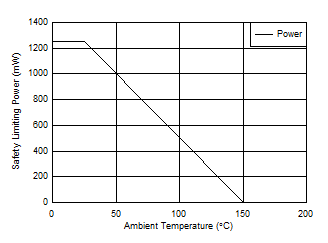Figure 3. Thermal Derating Curve for Safety Limiting Power per VDE

### 7.12 Typical Characteristics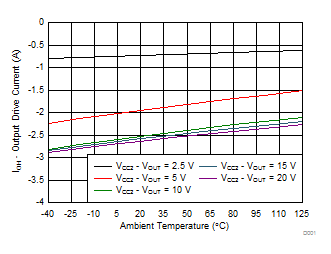Figure 4. Output High Drive Current vs Temperature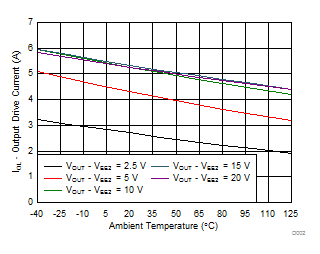Figure 6. Output Low Drive Current vs Temperature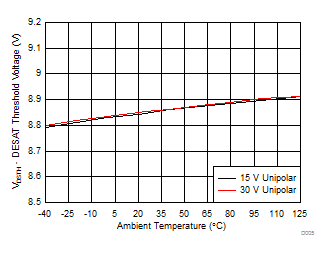Unipolar: VCC2 – VEE2 = VCC2 – GND2
Figure 8. DESAT Threshold Voltage vs Temperature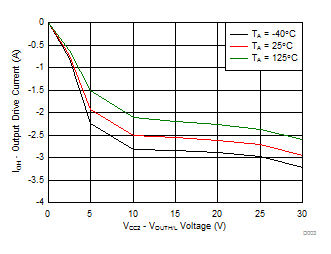Figure 5. Output High Drive Current vs Output Voltage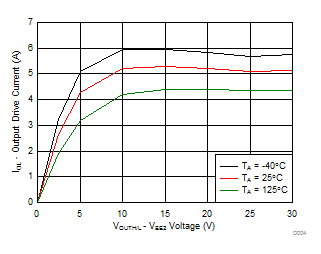Figure 7. Output Low Drive Current vs Output Voltage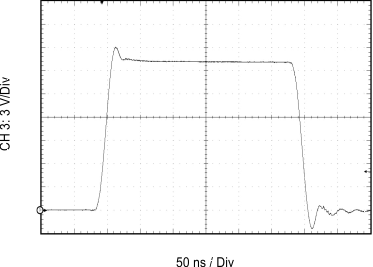CL = 1 nF RGH = 0 Ω RGL = 0 Ω VCC2 – VEE2 = VCC2 – GND2 = 20 V
Figure 9. Output Transient Waveform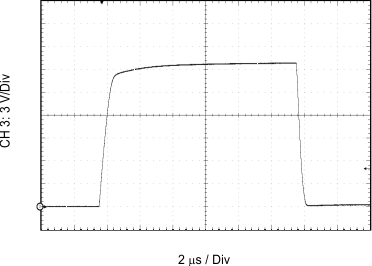CL = 100 nF RGH = 0 Ω RGL = 0 Ω VCC2 – VEE2 = VCC2 – GND2 = 20 V
Figure 11. Output Transient Waveform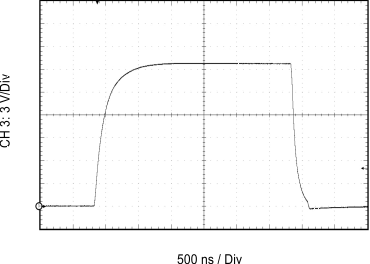CL = 10 nF RGH = 10 Ω RGL = 5 Ω VCC2 – VEE2 = VCC2 – GND2 = 20 V
Figure 13. Output Transient Waveform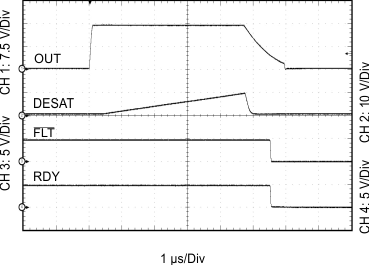CL = 10 nF RGH = 0 Ω RGL = 0 Ω VCC2 – VEE2 = VCC2 – GND2 = 15 V DESAT = 220 pF
Figure 15. Output Transient Waveform DESAT, RDY, and FLTCL = 10 nF RGH = 0 Ω RGL = 0 Ω VCC2 – VEE2 = VCC2 – GND2 = 30 V DESAT = 220 pF
Figure 17. Output Transient Waveform DESAT, RDY, and FLT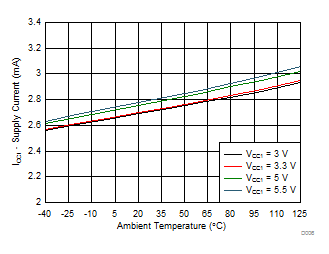IN+ = High IN– = Low
Figure 19. ICC1 Supply Current vs Temperature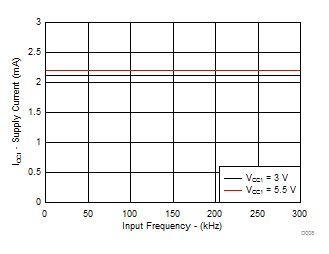Figure 21. ICC1 Supply Current vs Input Frequency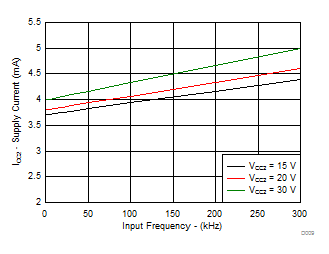No CL
Figure 23. ICC2 Supply Current vs Input Frequency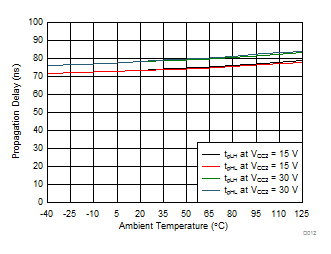CL = 1 nF RGH = 0 Ω RGL = 0 Ω VCC1 = 5 V
Figure 25. Propagation Delay vs Temperature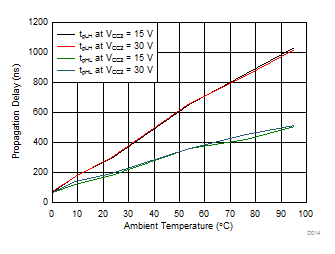RGH = 10 Ω RGL = 5 Ω VCC1 = 5 V
Figure 27. Propagation Delay vs Load Capacitance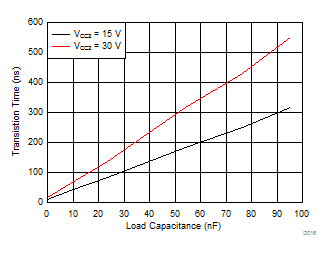RGH = 0 Ω RGL = 0 Ω VCC1 = 5 V
Figure 29. tf Fall Time v. Load Capacitance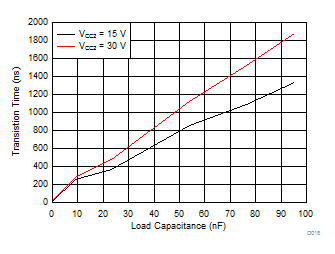RGH = 10 Ω RGL = 5 Ω VCC1 = 5 V
Figure 31. tf Fall Time vs Load Capacitance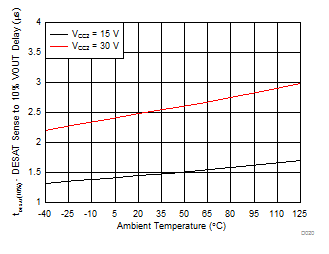CL = 10 nF RGH = 0 Ω RGL = 0 Ω
Figure 33. DESAT Sense to VOUT 10% Delay vs Temperature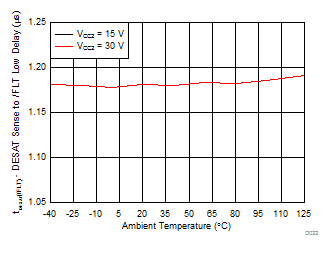Figure 35. DESAT Sense to Fault Low Delay vs Temperature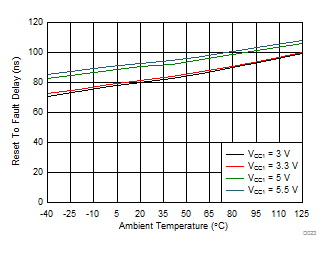Figure 37. Reset to Fault Delay Across Temperature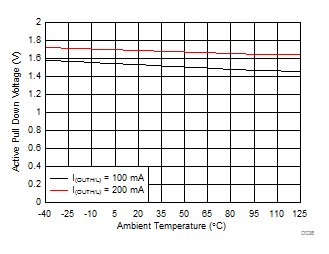Figure 39. Active Pulldown Voltage vs Temperature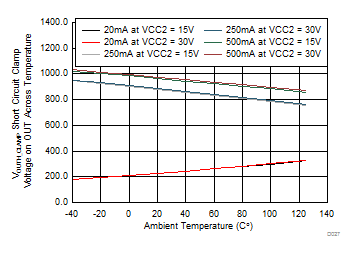Figure 41. VOUTH_CLAMP - Short-Circuit Clamp Voltage on OUTH Across Temperature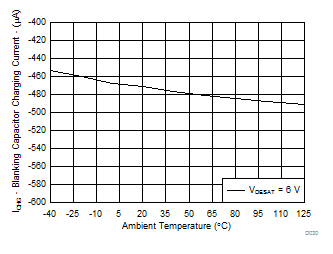VCC2 = 15 V DESAT = 6 V
Figure 43. Blanking Capacitor Charging Current vs Temperature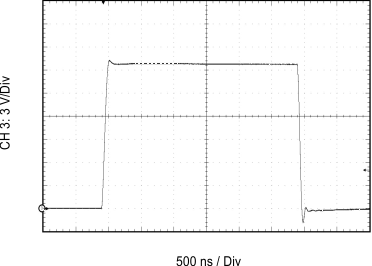CL = 10 nF RGH = 0 Ω RGL = 0 Ω VCC2 – VEE2 = VCC2 – GND2 = 20 V
Figure 10. Output Transient Waveform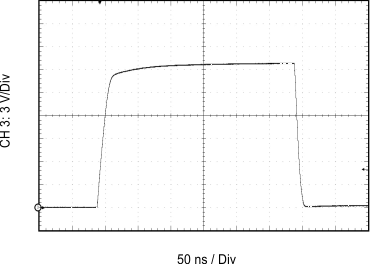CL = 1 nF RGH = 10 Ω RGL = 5 Ω VCC2 – VEE2 = VCC2 – GND2 = 20 V
Figure 12. Output Transient Waveform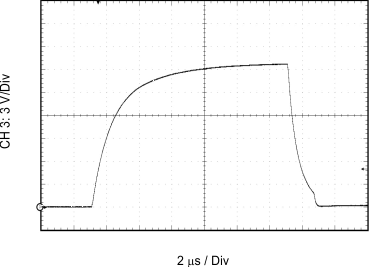CL = 100 nF RGH = 10 Ω RGL = 5 Ω VCC2 – VEE2 = VCC2 – GND2 = 20 V
Figure 14. Output Transient Waveform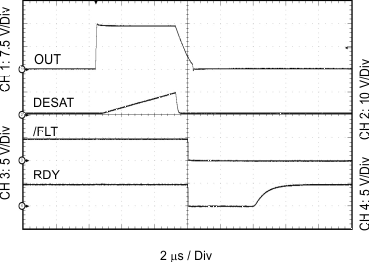CL = 10 nF RGH = 0 Ω RGL = 0 Ω VCC2 – VEE2 = VCC2 – GND2 = 15 V DESAT = 220 pF
Figure 16. Output Transient Waveform DESAT, RDY, and FLT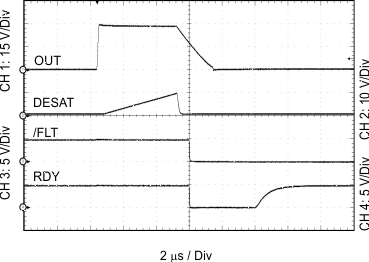CL = 10 nF RGH = 0 Ω RGL = 0 Ω VCC2 – VEE2 = VCC2 – GND2 = 30 V DESAT = 220 pF
Figure 18. Output Transient Waveform DESAT, RDY, and FLT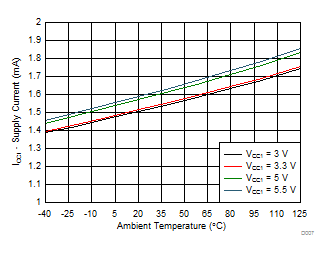IN+ = Low IN– = Low
Figure 20. ICC1 Supply Current vs Temperature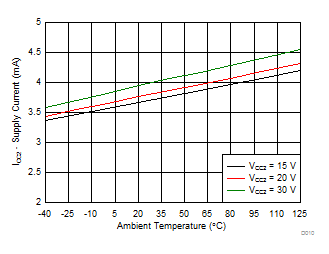Input frequency = 1 kHz
Figure 22. ICC2 Supply Current vs Temperature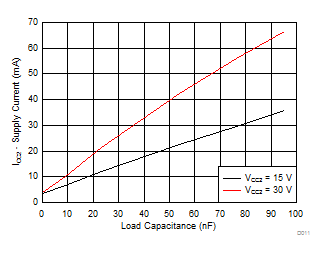RGH = 10 Ω RGL = 5 Ω, 20 kHz
Figure 24. ICC2 Supply Current vs Load Capacitance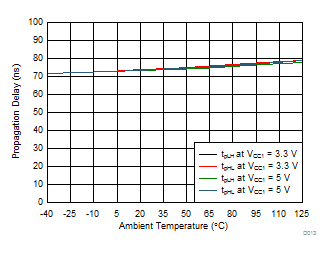CL = 1 nF RGH = 0 Ω RGL = 0 Ω VCC2 = 15 V
Figure 26. Propagation Delay vs Temperature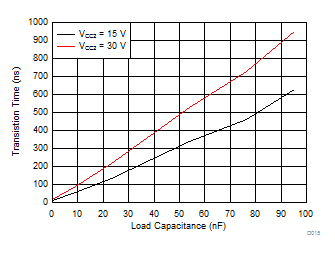RGH = 0 Ω RGL = 0 Ω VCC1 = 5 V
Figure 28. tr Rise Time vs Load Capacitance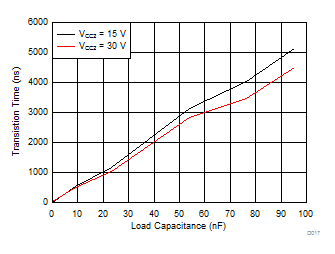RGH = 10 Ω RGL = 5 Ω VCC1 = 5 V
Figure 30. tr Rise Time vs Load Capacitance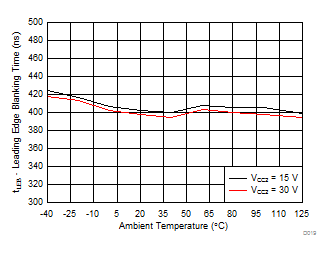Figure 32. Leading Edge Blanking Time With Temperature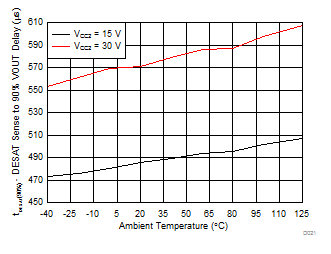CL = 10 nF RGH = 0 Ω RGL = 0 Ω
Figure 34. DESAT Sense to VOUT 90% Delay vs Temperature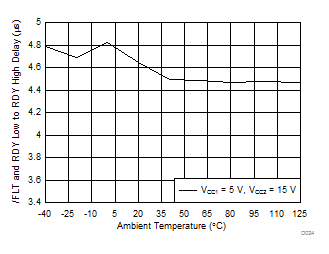Figure 36. Fault and RDY Low to RDY High Delay vs Temperature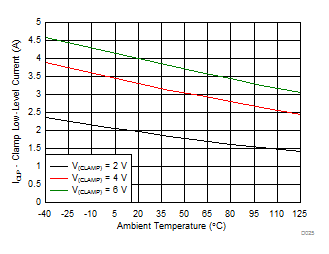Figure 38. Miller Clamp Current vs Temperature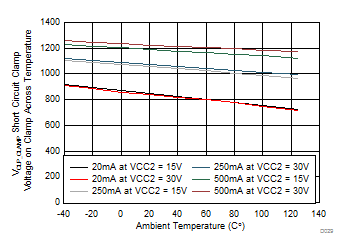Figure 40. VCLP_CLAMP - Short-Circuit Clamp Voltage on Clamp Across Temperature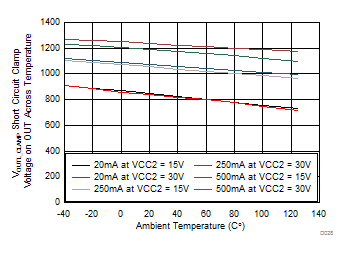Figure 42. VOUTL_CLAMP - Short-Circuit Clamp Voltage on OUTL Across Temperature